Courses

# Modulus and Conjugate of a Complex Number Mathematics Notes | EduRev

## Algebra for IIT JAM Mathematics

Created by: Veda Institute

## Mathematics : Modulus and Conjugate of a Complex Number Mathematics Notes | EduRev

The document Modulus and Conjugate of a Complex Number Mathematics Notes | EduRev is a part of the Mathematics Course Algebra for IIT JAM Mathematics.
All you need of Mathematics at this link: Mathematics

Conjugate of A Complex Number
Complex numbers are represented in a binomial form as (a + ib). It almost invites you to play with that ‘+’ sign. What happens if we change it to a negative sign? Let z = a + ib be a complex number. We define another complex number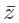such that= a – ib. We callor the complex number obtained by changing the sign of the imaginary part (positive to negative or vice versa), as the conjugate of z. Let us now find the product z= (a + ib)×(a – ib)
Hence, z= {a2 -i(ab) + i(ab) + b2} = (a2 + b2)…(1)
If a and b are large numbers, the sum in (1) will be greater. So one can use this equation to measure the value of a complex number.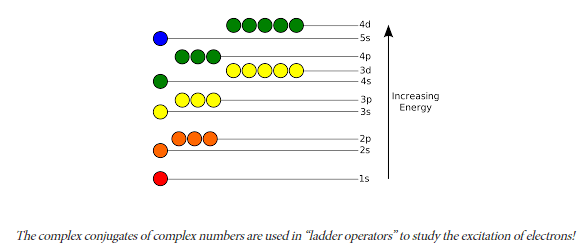Modulus of A Complex Number
There is a way to get a feel for how big the numbers we are dealing with are. We take the complex conjugate and multiply it by the complex number as done in (1). Hence, we define the product z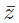as the square of the Absolute value or modulus of a complex number. Therefore, we can write z=|z|2
This is done because as we just discussed, zgives a way to measure the Absolute value or magnitude of our complex number. The actual reason for this definition will get clear once you learn about the Argand Plane.
Therefore, |z2 = (a2 + b2 ) [Using (1)]
Hence, |z| =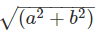…(2)
The above equation represents the modulus or the absolute value of our complex number z.

Important Identities
• If |z|2 = 1 = |z|, i.e. z is a complex number of unit modulus, then from (1), we have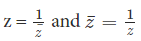• Also If z1 and z2 is any complex number, we have the following identities:
|z1 z2| = |z1| |z2|
•Modulus (z1 / z2)= Modulus of z1 /Modulus of z2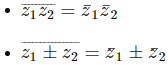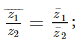with the accord that z2 ≠ 0

Solved Examples For You
Example 1: If z = 2 – i, find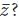?
Solution: All we need to do is to change the sign of the imaginary term. Therefore, we denote it as follows:z = 2 – i or (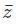)= (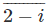)Now ¯ or ‘bar’ or ‘dagger’ becomes an operation that transforms the imaginary part of a complex number in sign. In other words, we can write: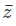= 2 + i. Note that there is no change in the sign of 2.

Example 2: If z = a+ib, show that |z2 = z.
Solution: We know that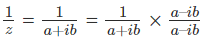Therefore, we have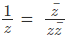[using the definition of conjugate]
Hence,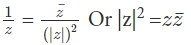Example 3: The maximum value of ∣z∣ when z satisfies the condition ∣z+ 2/z ∣ = 2 is:
A) 1
B) √3+1
C) √3–1
D) -1
Solution: (B) We have the condition that modulus (z+2/z) = 2. Therefore, can we not treat this as an equation in one variable? We can but the equation would be second order in z and we won’t be able to get a relation for |z| alone. So we do the following:
|z| can be written as =|z+[2/z]–[2/z]|≤|z+[2/z]|≤|z+[2/z]|+ 2/|z|
Therefore,|z|≤2+2/|z| Or |z|≤(2|z|+2)/|z|
Hence,|z|2 ≤ 2|z|+2 Or |z|2 – 2|z| ≤ 2
To get the value of |z|, let’s convert the LHS of the above equation into a perfect square. After adding 1 on both the side, we have |z|2–2|z|(1)+(1)2 ≤  2 +(1)2
(|z| – 1)2 ≤ 3
Therefore,–√3 ≤ (|z| – 1) ≤ √3
Hence, the maximum value of |z| will be √3 + 1

Offer running on EduRev: Apply code STAYHOME200 to get INR 200 off on our premium plan EduRev Infinity!

,

,

,

,

,

,

,

,

,

,

,

,

,

,

,

,

,

,

,

,

,

;